# 快速掌握Java8 Stream函数式编程技巧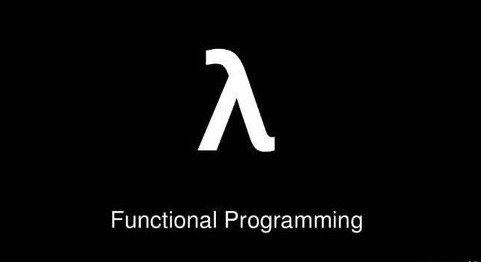## 函数式编程优势

• “函数第一位”，即函数可以出现在任何地方。
• 可以把函数作为参数传递给另一个函数，还可以将函数作为返回值。
• 让代码的逻辑更清晰更优雅。
• 减少了可变量(Immutable Variable)的声明，程序更为安全。
• 支持惰性计算。

## Lambda语法三部分

• 一个括号内用逗号分隔的形式参数，参数是函数式接口里面方法的参数
• 一个箭头符号：->
• 方法体，可以是表达式和代码块，方法体函数式接口里面方法的实现，如果是代码块，则必须用{}来包裹起来，且需要一个return 返回值，但有个例外，若函数式接口里面方法返回值是void，则无需{}。例如：
• (parameters) -> expression 或者 (parameters) -> { statements; }

ObjectReference::methodName

• 如果是静态方法，则是ClassName::methodName。如 Object ::equals
• 如果是实例方法，则是Instance::methodName。如Object obj=new Object();obj::equals;
• 构造函数.则是ClassName::new

## Stream是什么

Stream是Java8中新加入的api，更准确的说: Java 8 中的 Stream 是对集合（Collection）对象功能的增强，它专注于对集合对象进行各种非常便利、高效的聚合操作，或者大批量数据操作 。Stream API 借助于同样新出现的 Lambda 表达式，极大的提高编程效率和程序可读性。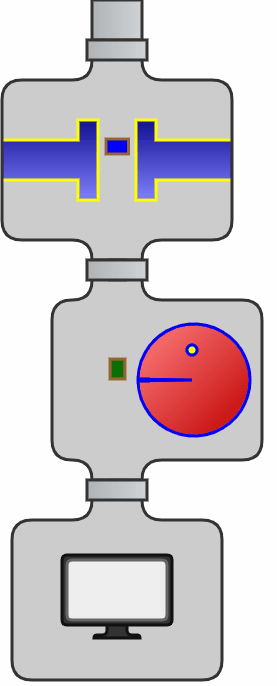## Stream操作分类

Stream上的所有操作分为两类：中间操作和结束操作，中间操作只是一种标记，只有结束操作才会触发实际计算。中间操作又可以分为无状态的(Stateless)和有状态的(Stateful)，无状态中间操作是指元素的处理不受前面元素的影响，而有状态的中间操作必须等到所有元素处理之后才知道最终结果，比如排序是有状态操作，在读取所有元素之前并不能确定排序结果；结束操作又可以分为短路操作和非短路操作，短路操作是指不用处理全部元素就可以返回结果，比如找到第一个满足条件的元素。之所以要进行如此精细的划分，是因为底层对每一种情况的处理方式不同。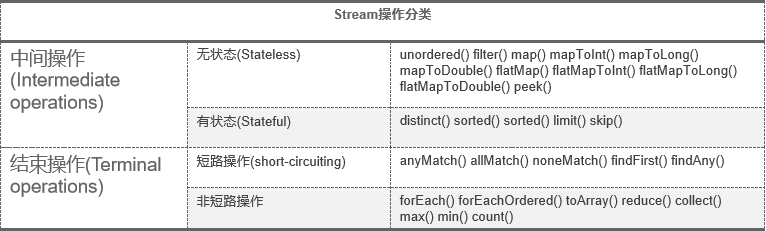## Stream API等价实现

int longest = 0;
for(String str : strings){
if(str.startsWith("A")){// 1. filter(), 保留以A开头的字符串
int len = str.length();// 2. mapToInt(), 转换成长度
longest = Math.max(len, longest);// 3. max(), 保留最长的长度
}
}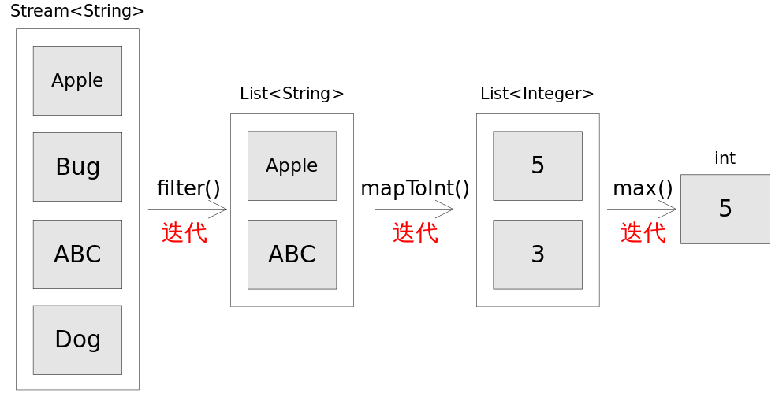int longest = strings.stream()
.filter(str -> str.startsWith("A"))
.mapToInt(str ->  str.length())
//.mapToInt(String::length)
.max();

## Stream串行与并行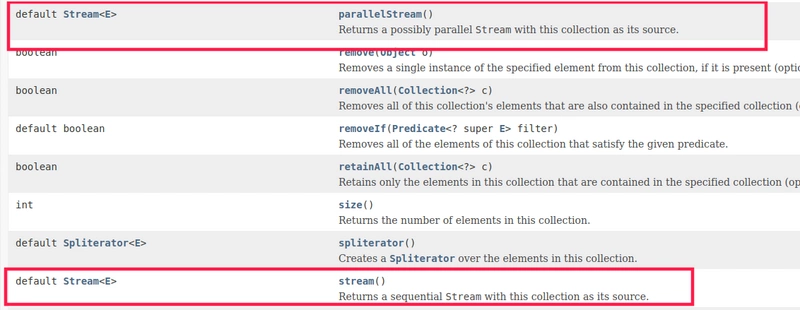Stream可以分为串行与并行两种，串行流和并行流差别就是单线程和多线程的执行。 default Stream stream() ： 返回串行流 default Stream parallelStream() ： 返回并行流 stream()和parallelStream()方法返回的都是java.util.stream.Stream<E>类型的对象，说明它们在功能的使用上是没差别的。唯一的差别就是单线程和多线程的执行。

## Stream性能总结

1.对于简单操作，比如最简单的遍历，Stream串行API性能明显差于显示迭代，但并行的Stream API能够发挥多核特性。

2.对于复杂操作，Stream串行API性能可以和手动实现的效果匹敌，在并行执行时Stream API效果远超手动实现。

• 对于简单操作推荐使用外部迭代手动实现。
• 对于复杂操作，推荐使用Stream API。
• 在多核情况下，推荐使用并行Stream API来发挥多核优势。
• 单核情况下不建议使用并行Stream API。 如果出于代码简洁性考虑，使用Stream API能够写出更短的代码。即使是从性能方面说，尽可能的使用Stream API也另外一个优势，那就是只要Java Stream类库做了升级优化，代码不用做任何修改就能享受到升级带来的好处。

## Stream 来源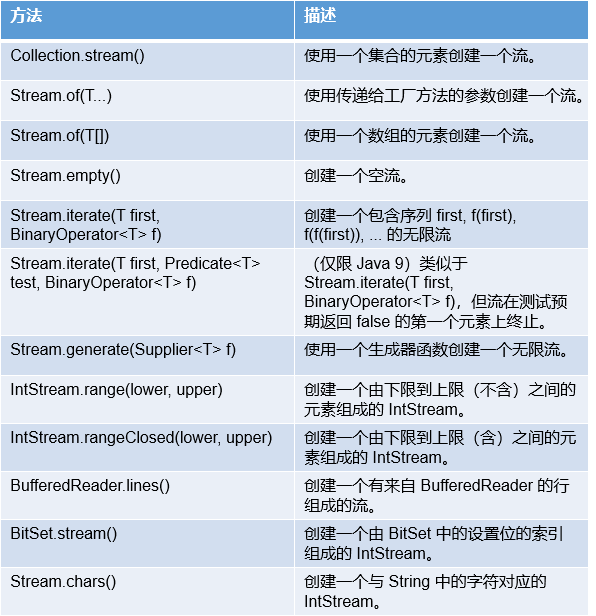## Stream 操作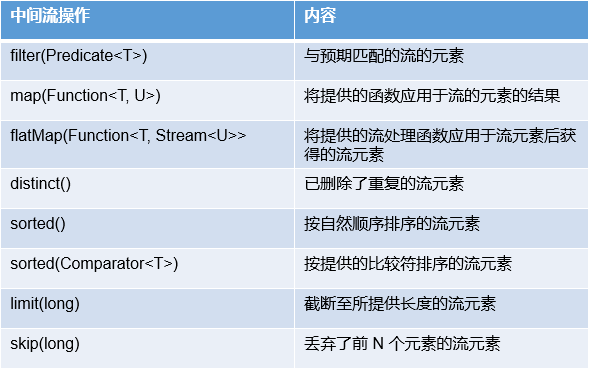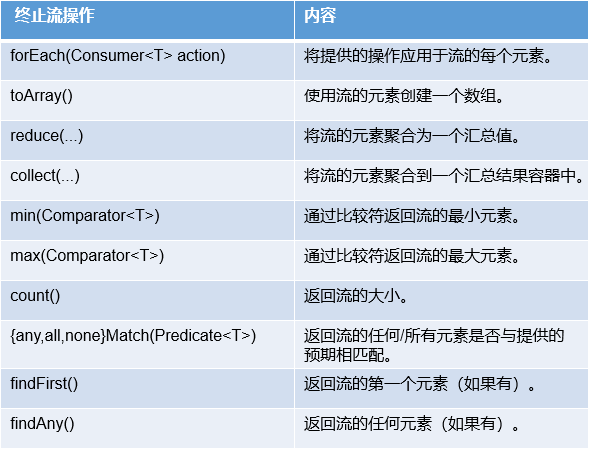## Stream 流与集合比较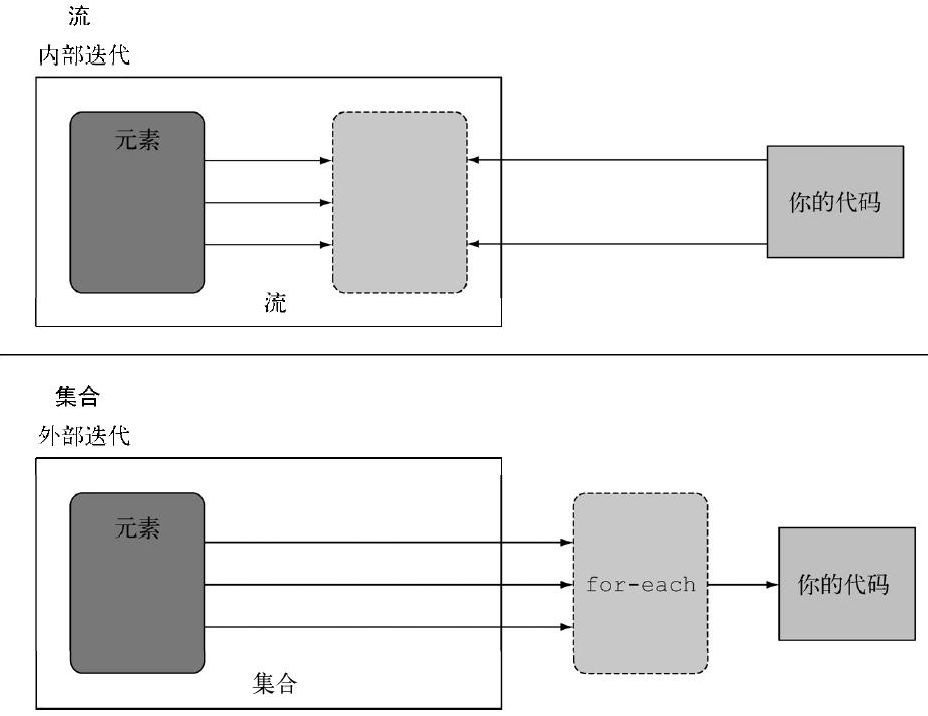## Stream 基本使用

filter筛选（中间操作）

List<Integer> integerList = Arrays.asList(1, 1, 2, 3, 4, 5);

Stream<Integer> stream = integerList.stream().filter(i -> i > 3);

distinct去重（中间操作）

List<Integer> integerList = Arrays.asList(1, 1, 2, 3, 4, 5);

Stream<Integer> stream = integerList.stream().distinct();

limit限制（中间操作）

 List<Integer> integerList = Arrays.asList(1, 1, 2, 3, 4, 5);

Stream<Integer> stream = integerList.stream().limit(3);

skip跳过（中间操作）

 List<Integer> integerList = Arrays.asList(1, 1, 2, 3, 4, 5);

Stream<Integer> stream = integerList.stream().skip(2);

map流映射（中间操作）

List<String> stringList = Arrays.asList("Java 8", "Lambdas",  "In", "Action");

Stream<Integer> stream = stringList.stream().map(String::length);

flatMap流转换（中间操作）

List<List<Integer>> lists = new ArrayList<List<Integer>>() {{
}};

Stream<Integer> stream = lists.stream().flatMap(List::stream);

//Stream<Integer> stream = lists.stream().flatMap(list -> list.stream());

allMatch匹配所有（中间操作）

List<Integer> integerList = Arrays.asList(1, 2, 3, 4, 5);

if (integerList.stream().allMatch(i -> i > 3)) {
System.out.println("值都大于3");
}

noneMatch全部不匹配（中间操作）

List<Integer> integerList = Arrays.asList(1, 2, 3, 4, 5);

if (integerList.stream().noneMatch(i -> i > 3)) {
System.out.println("值都小于3");
}

anyMatch匹配其中一个（中间操作）

List<Integer> integerList = Arrays.asList(1, 2, 3, 4, 5);
if (integerList.stream().anyMatch(i -> i > 3)) {
System.out.println("存在大于3的值");
}

findFirst查找第一个（终端操作）

List<Integer> integerList = Arrays.asList(1, 2, 3, 4, 5);
Optional<Integer> result = integerList.stream().filter(i -> i > 3).findFirst();

findAny随机查找一个（终端操作）

List<Integer> integerList = Arrays.asList(1, 2, 3, 4, 5);
if (integerList.stream().findAny(i -> i > 3)) {
System.out.println("存在大于3的值");
}

## Stream 常用统计

List<Integer> ints = Arrays.asList(1, 1, 2, 2, 3);
//统计流中元素个数
ints.stream().count();
ints.stream().collect(Collectors.counting());

//获取流中最小值
ints.stream().min(Integer::compareTo);
ints.stream().collect(Collectors.minBy(Integer::compareTo));

//获取流中最大值
ints.stream().max(Integer::compareTo);
ints.stream().collect(Collectors.maxBy(Integer::compareTo));

//求和
ints.stream().mapToInt(Integer::intValue).sum();
ints.stream().collect(Collectors.summingInt(Integer::intValue));
ints.stream().reduce(0, Integer::sum);

//平均值
ints.stream().collect(Collectors.averagingInt(Integer::intValue));

//通过summarizingInt同时求总和、平均值、最大值、最小值
ints.stream().collect(Collectors.summarizingInt(Integer::intValue));

## Stream 终端操作（collect）

List<Integer> ints = Arrays.asList(1, 1, 2, 2, 3);
//返回List
ints.stream().collect(Collectors.toList());

//返回Set
ints.stream().collect(Collectors.toSet());

//返回Map
ints.stream().collect(Collectors.toMap(k -> k, v -> v, (v1, v2) -> v1));

//group分组
ints.stream().collect(Collectors.groupingBy(k -> k));

//partitioningBy分区
ints.stream().collect(Collectors.partitioningBy(k -> k % 2 == 0));

## Stream参考文献

posted @ 2020-10-18 15:43  胡峻峥  阅读(133)  评论(0编辑  收藏